# 算法纲要

## HashMap

• 默认长度16
• 根据key的二进制，与上size - 1（1111）作为index(所以时间复杂度1)
• 相同的index，做成单向链表，新的作为头，get的时候按顺序比较key
• 负载因子75%，大于这个需要resize（乘以2倍），过程不线程安全，高并发插入可能会引起循环链表

## 红黑树

• 二叉树查找，左小右大，缺点是插入的时候，深度可能太大
• 红黑树的规则，控制了平衡，最深的不超过最浅的2倍，时间复杂度O(lgn), n个节点, 最高2log(n+1)
• 通过切换黑、红颜色，左旋、右旋的方式确保插入、删除符合规则，控制平衡

## 循环链表

hash Map的方式判断 O(n) O(n)

## 动态规划

• F(n) = F(n-1) + F(n-2), 写出来是一个递归， 递归是一个树结构, 时间复杂度是O(2^n)
• 由于F(n-1)在下一步F(n-2)算过， 使用Hash Map可以存储（备忘录算法）时间复杂度O(n), 空间复杂度O(n)
• 倒过来F(3) = F(2) + F(1), 只用两个零时变量即可算F(n), 空间复杂度O(1)
``````func someSum(n: Int) -> Int {
guard n > 0 else { return 0 }
let a = 1
let b = 2
guard n == 1 else { return a }
guard n == 2 else { return b }
return someSum(n-1) + someSum(n-2) // F(n) = F(n-1) + F(n-2)
}
``````
``````func someSum(n: Int, inout map: [Int: Int]) -> Int {
guard n > 0 else { return 0 }
let a = 1
let b = 2
guard n == 1 else { return a }
guard n == 2 else { return b }
if let some = map[n] {
return some
}
let sum = someSum(n-1) +someSum(n-2)
map[n] = sum
return sum
}
``````
``````func someSum(n: Int) {
guard n > 0 else { return 0 }
var a = 1
var b = 2
guard n == 1 else { return a }
guard n == 2 else { return b }
var temp = 0
for i in 3..<n {
temp = a + b    // F(n+2) = F(n) + F(n+1)
a = b            // F(n) = F(n+1)
b = temp        // F(n+1) = F(n+2)
}
return temp
}
``````

• 数学原理
• 计算机原理问题优化掉

## 排序算法

• 选择排序

1. 暂定第一个元素为最小元素，往后遍历，逐个与最小元素比较，若发现更小者，与先前的"最小元素"交换位置。达到更新最小元素的目的。
2. 一趟遍历完成后，能确保刚刚完成的这一趟遍历中，最的小元素已经放置在前方了。然后缩小排序范围，新一趟排序从数组的第二个元素开始。
3. 在新一轮排序中重复第1、2步骤，直到范围不能缩小为止，排序完成。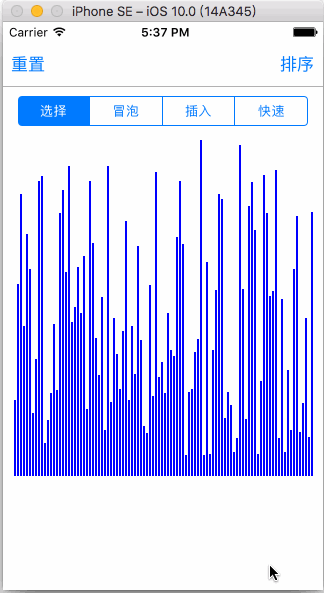• 冒泡排序

1. 在一趟遍历中，不断地对相邻的两个元素进行排序，小的在前大的在后，这样会造成大值不断沉底的效果，当一趟遍历完成时，最大的元素会被排在后方正确的位置上。
2. 然后缩小排序范围，即去掉最后方位置正确的元素，对前方数组进行新一轮遍历，重复第1步骤。直到范围不能缩小为止，排序完成。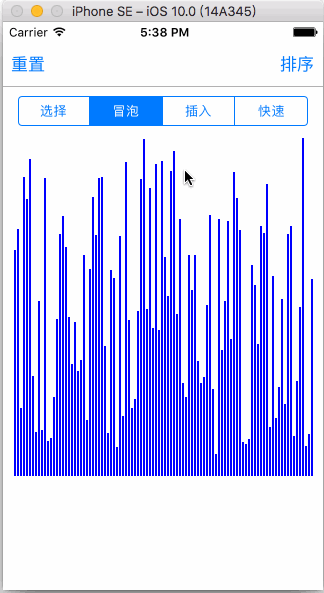• 插入排序

• 从一个乱序的数组中依次取值，插入到一个已经排好序的数组中

• 开始时前方有序区只有一个元素，就是数组的第一个元素。然后把从第二个元素开始直到结尾的数组作为乱序区。

• 从乱序区取第一个元素，把它正确插入到前方有序区中。把它与前方无序区的最后一个元素比较，亦即与它的前一个元素比较。
• 如果比前一个元素要大，则不需要交换，这时有序区扩充一格，乱序区往后缩减一格，相当于直接拼在有序区末尾。
• 如果和前一个元素相等，则继续和前二元素比较、再和前三元素比较......如果往前遍历到头了，发现前方所有元素值都长一个样的话(囧)，那也可以，不需要交换，这时有序区扩充一格，乱序区往后缩减一格，相当于直接拼在有序区末尾。如果比前一个元素大呢？对不起作为有序区不可能出现这种情况。如果比前一个元素小呢，请看下一点。
• 如果比前一个元素小，则交换它们的位置。交换完后，继续比较取出元素和它此时的前一个元素，若更小就交换，若相等就比较前一个，直到遍历完成。
• 往后缩小乱序区范围，继续取缩小范围后的第一个元素，重复第2步骤。直到范围不能缩小为止，排序完成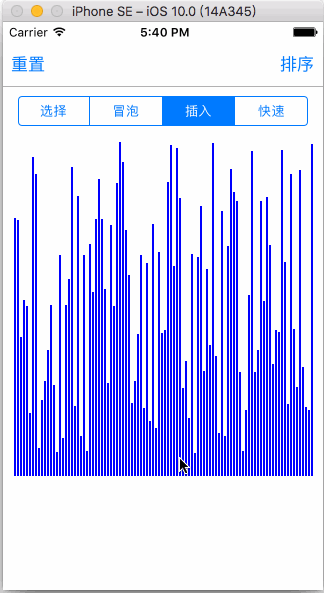• 快速排序 平均Ο(nlogn),最坏Ο(n2)

• 原始的快排。
• 为制造适合高效排序环境而事先打乱数组顺序的快排。
• 为数组内大量重复值而优化的三向切分快排。

• 从数列中挑出一个元素，称为 “基准”（pivot），

• 重新排序数列，所有元素比基准值小的摆放在基准前面，所有元素比基准值大的摆在基准的后面（相同的数可以到任一边）。在这个分区退出之后，该基准就处于数列的中间位置。这个称为分区（partition）操作。
• 递归地（recursive）把小于基准值元素的子数列和大于基准值元素的子数列排序。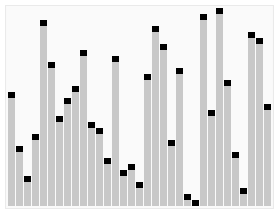• 堆排序 平均Ο(nlogn)

原理上是一颗完全二叉树参考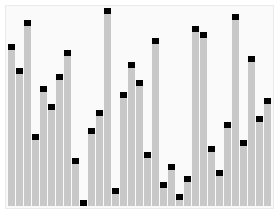• 归并排序O(nlogn)

1. 先逐步拆分成最小单元
2. 然后按顺序合并小单元，小单元合并成大单元## 查找算法

• 二分查找算法
• 在有序数组中查找某一特定元素的搜索算法
• BFPRT(线性查找算法)

• DFS（深度优先搜索）
• BFS(广度优先搜索)
• Dijkstra算法

• 朴素贝叶斯分类算法